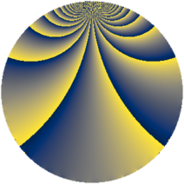# Properties

 Label 1089.1.sLevel $1089$ Weight $1$ Character orbit 1089.s Rep. character $\chi_{1089}(40,\cdot)$ Character field $\Q(\zeta_{30})$ Dimension $24$ Newform subspaces $2$ Sturm bound $132$ Trace bound $1$

# Related objects

## Defining parameters

 Level: $$N$$ $$=$$ $$1089 = 3^{2} \cdot 11^{2}$$ Weight: $$k$$ $$=$$ $$1$$ Character orbit: $$[\chi]$$ $$=$$ 1089.s (of order $$30$$ and degree $$8$$) Character conductor: $$\operatorname{cond}(\chi)$$ $$=$$ $$99$$ Character field: $$\Q(\zeta_{30})$$ Newform subspaces: $$2$$ Sturm bound: $$132$$ Trace bound: $$1$$

## Dimensions

The following table gives the dimensions of various subspaces of $$M_{1}(1089, [\chi])$$.

Total New Old
Modular forms 120 88 32
Cusp forms 24 24 0
Eisenstein series 96 64 32

The following table gives the dimensions of subspaces with specified projective image type.

$$D_n$$ $$A_4$$ $$S_4$$ $$A_5$$
Dimension 8 0 16 0

## Trace form

 $$24 q - q^{3} - q^{4} - 3 q^{5} + 3 q^{9} + O(q^{10})$$ $$24 q - q^{3} - q^{4} - 3 q^{5} + 3 q^{9} - 12 q^{12} + 4 q^{14} + q^{15} - q^{16} + q^{20} - 8 q^{23} + 2 q^{27} + q^{31} + 2 q^{36} + 6 q^{37} - 4 q^{42} - 24 q^{45} - 3 q^{47} - 6 q^{48} - q^{49} - 2 q^{53} - 4 q^{58} + q^{59} + 6 q^{60} - 6 q^{64} + 12 q^{67} + 2 q^{69} - 4 q^{70} + 6 q^{71} - 2 q^{80} + 3 q^{81} + 16 q^{89} + 2 q^{92} - 3 q^{93} + q^{97} + O(q^{100})$$

## Decomposition of $$S_{1}^{\mathrm{new}}(1089, [\chi])$$ into newform subspaces

Label Dim $A$ Field Image CM RM Traces $q$-expansion
$a_{2}$ $a_{3}$ $a_{5}$ $a_{7}$
1089.1.s.a $8$ $0.543$ $$\Q(\zeta_{15})$$ $D_{3}$ $$\Q(\sqrt{-11})$$ None $$0$$ $$1$$ $$-1$$ $$0$$ $$q-\zeta_{30}^{7}q^{3}-\zeta_{30}^{13}q^{4}+\zeta_{30}q^{5}+\cdots$$
1089.1.s.b $16$ $0.543$ 16.0.$$\cdots$$.1 $S_{4}$ None None $$0$$ $$-2$$ $$-2$$ $$0$$ $$q+\beta _{1}q^{2}-\beta _{7}q^{3}+\beta _{2}q^{4}+(\beta _{3}+\beta _{15})q^{5}+\cdots$$